Like   Tweet   +1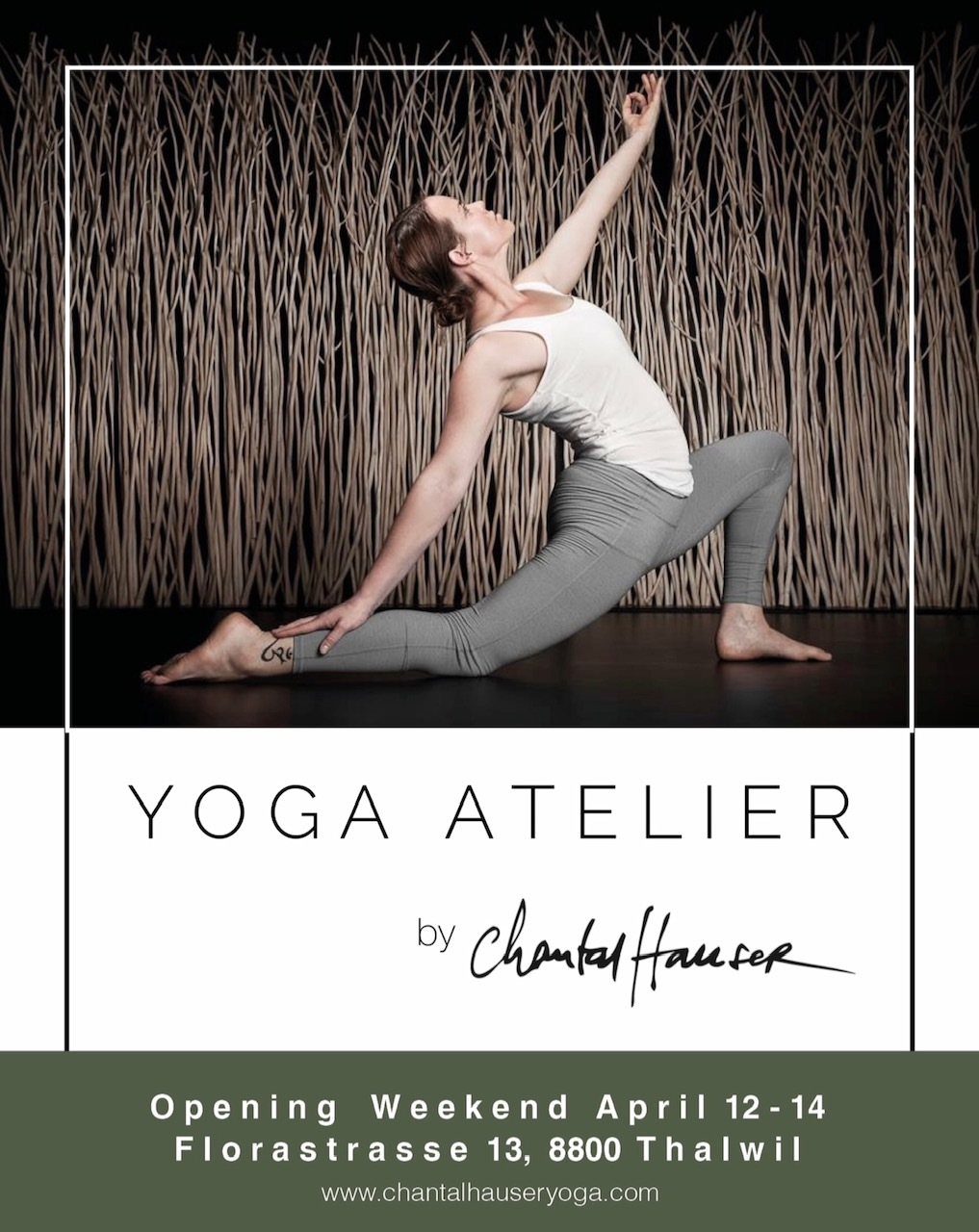## at the NEW Yoga Atelier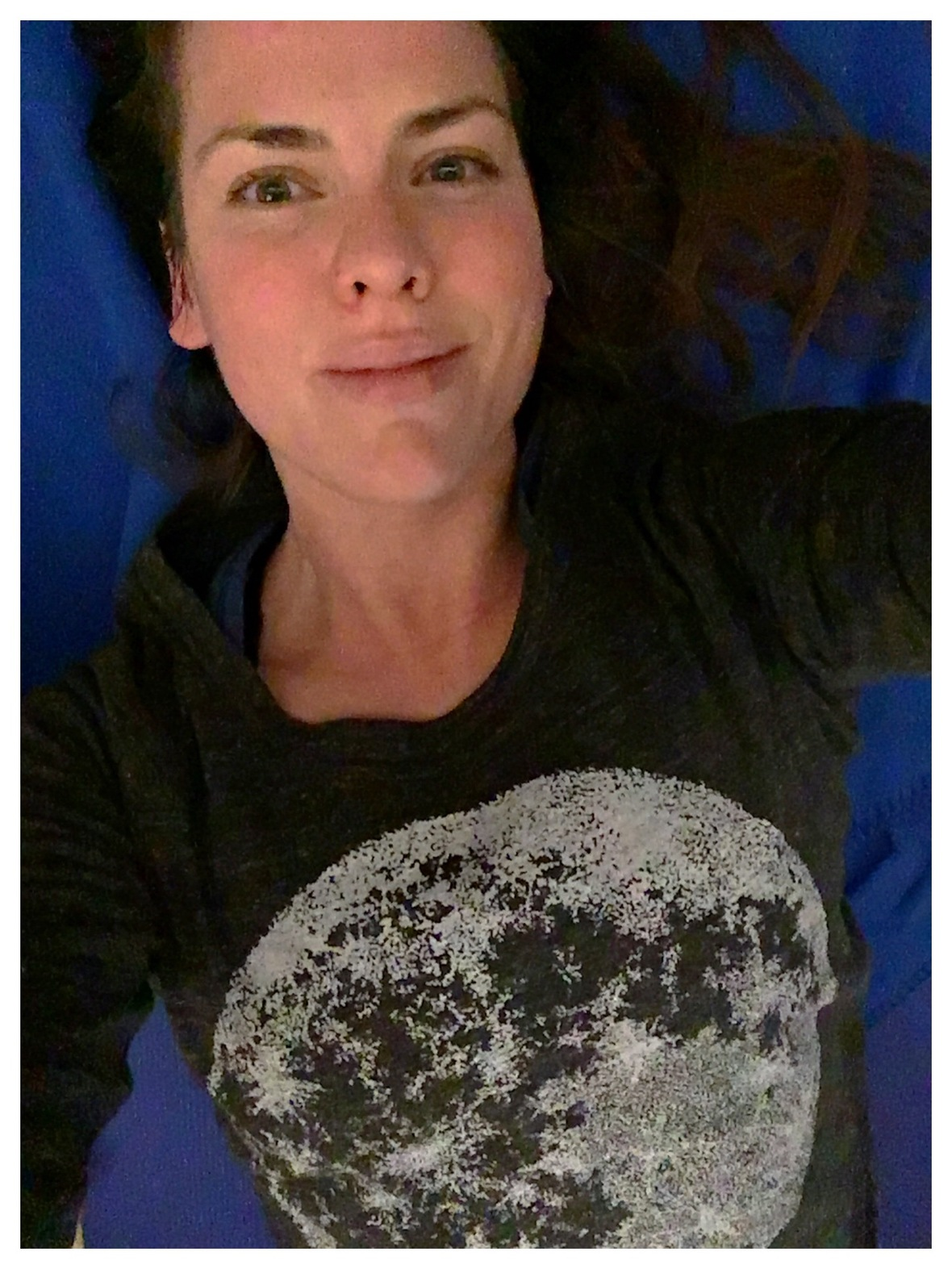Keep following your Dreams!

## Dear Yogi!

The Yoga Atelier has been open for a minute and today it started its 3rd week!

Wow! I don't even know where to start to tell you all that has happened within the last month. The day after Valentine's I signed the contract and "moved" in. It took me 4 long years of dreaming and many late night tears to get to this point. When I wrote you the last newsletter, I wasn't even sure if I'll get this space. Everything was up in the air.

Then, with the help of three awesome guys I was able to open the Yoga Atelier's doors within 2 weeks! We put in a new floor, painted the walls and ceiling. I also realized that picking out lights and curtains are not as easy as one thinks. We have worked tirelessly to make the Atelier ready to YOGA in!

If you have a dream, follow it! I did and I can say that it works if you work on it step by step. For the past 4 plus years, I bought something every month that I wanted to have in my Yoga Atelier. I had no idea where it will be, but I knew that it will be. I have a yoga journal that I use to write down ideas in, workshops, etc. I used it to write down everything I liked and disliked from yoga studios I knew and worked for. Therefore I am able to start without any crazy extra expenses, with somewhat of a plan and with a lot of space too feel the excitement!

I am still letting the feeling settle that I actually have a business location! I am currently floating on cloud 9, somewhere in my body and mind. There is still so much to do, but like tending a garden, one step at a time.

I am also absolutely thrilled to host my first Deep Immersion Training this upcoming weekend at the Atelier! It will be an amazing and precious time to spend a weekend immersing ourselves in yoga and all there is of us on the mat! I am very much excited for it and hope you are too!
(By the way, there is still one spot open if you want it!)

AND I want to THANK YOU if you came out and supported my dream come true on the very first day on Sunday, March 3!

There are many workshops and events, new classes and trainings coming up for you. Check out some of the sweet happenings below!

 table div table+table+table+table+table div table{width:100%;padding:0}table div table+table+table+table+table div table img{width:96.23%;padding:0;float:none}table div table+table+table+table+table div table td{width:100%;padding:0 1.88% 18px}/* styles */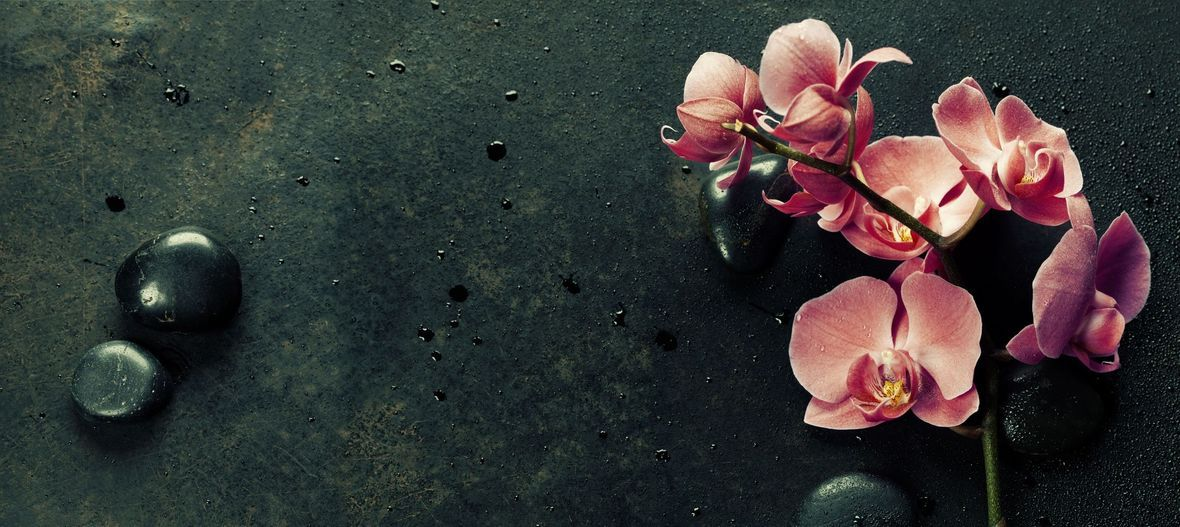/* styles */ See you on the mat! Much Love + Peace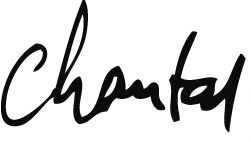## P.S.

Have you signed up for the Yoga Atelier OPENING WEEKEND yet?
Space is limited!

 table div table+table+table+table+table+table+table+table+table+table div table{width:100%;padding:0}table div table+table+table+table+table+table+table+table+table+table div table img{width:96.23%;padding:0;float:none}table div table+table+table+table+table+table+table+table+table+table div table td{width:100%;padding:0 1.88% 18px}/* styles */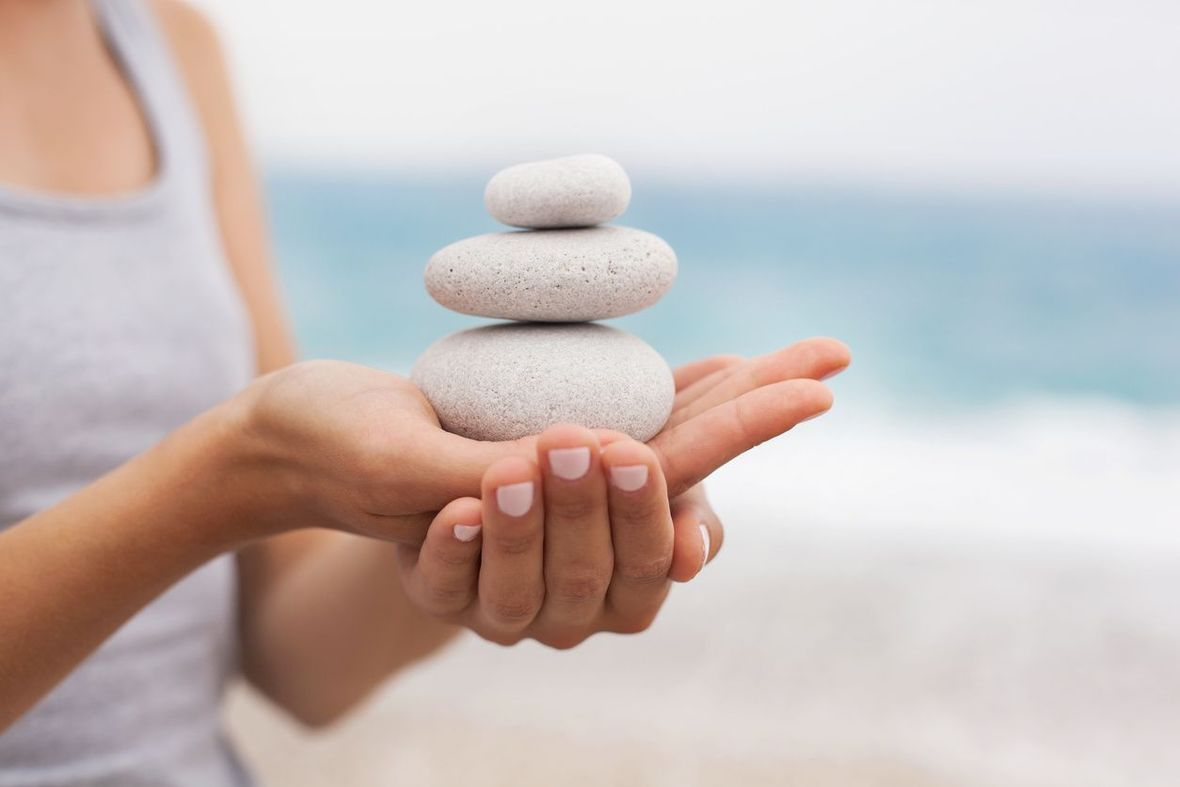## Group Schedule at the Yoga Atelier

Mondays
09:15 - 10:45 Monday Chill Flow (E)
12:15 - 13:00 Office Bewegung (CH)
19:00 - 20:30 Forrest Yoga (E)

Wednesdays
09:30 - 10:45 Morning Yoga (E) (Kids Welcome!)
17:00 - 18:00 Teen Yoga (E/CH) (10-14 yrs)
18:30 - 20:00 Hanuman Flow (E)

Thursdays
12:15 - 13:00 Office Bewegung (CH) mit Pascale (planned for May/June)
18:30 - 20:00 Forrest Yoga (CH) mit Pascale (Start am 4. April)

Sundays
10:30 - 11:45 Smooth Sunday (E/CH) (starts mid May) mit Pascale / Chantal
16:30 - 18:00 Hanuman Flow (E)

 table div table+table+table+table+table+table+table+table+table+table+table+table+table div table{width:100%;padding:0}table div table+table+table+table+table+table+table+table+table+table+table+table+table div table img{width:96.23%;padding:0;float:none}table div table+table+table+table+table+table+table+table+table+table+table+table+table div table td{width:100%;padding:0 1.88% 18px}/* styles */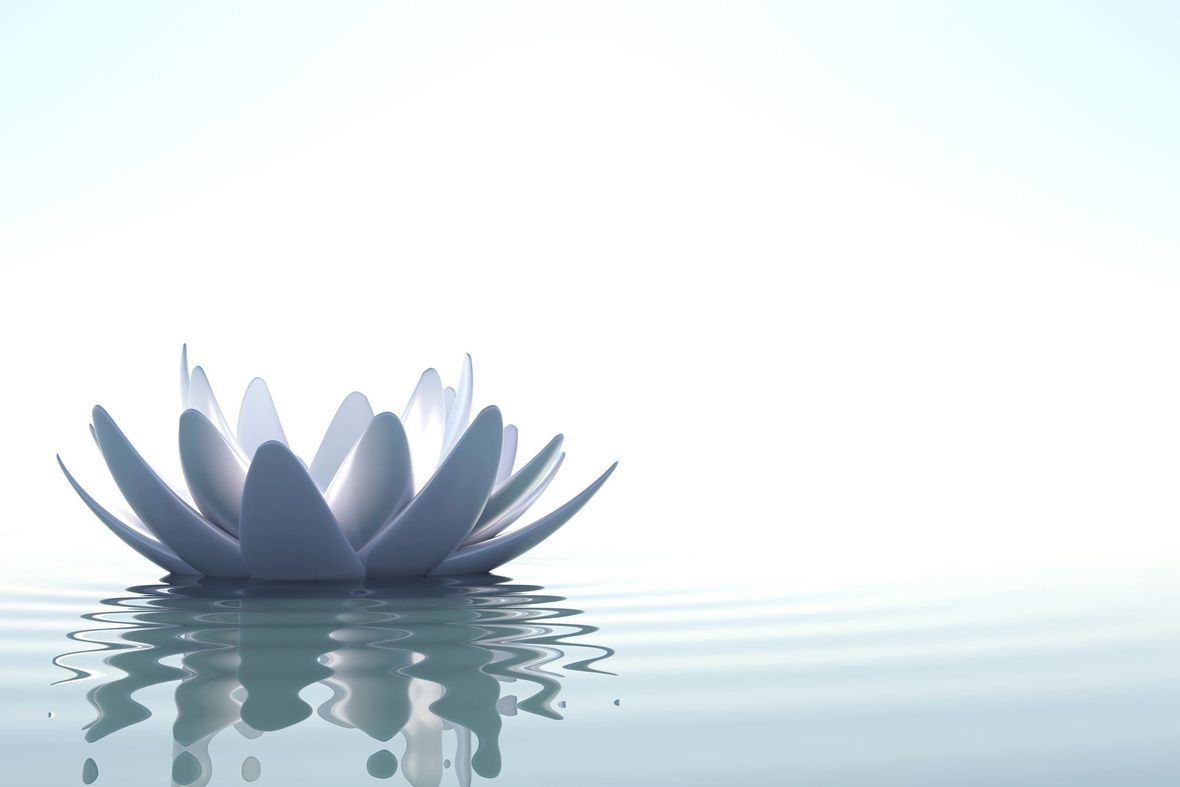## Investments at the Yoga Atelier

First Class 35.-

Fresh Pass 128.- 4 classes in 4 weeks

Drop In 45.- | Children 25.- | Lunch Yoga 25.-

Curious 5x for 42.- per class | (210.- valid for 8 weeks)
Cool 7x for 40.- per class | (280.- valid for 10 weeks)
Committed 10x for 38.- per class | (380.- valid for 12 weeks)
The Stardust 20x for 35.- per class | (700.- valid for 24 weeks)

 table div table+table+table+table+table+table+table+table+table+table+table+table+table+table+table div table{width:100%;padding:0}table div table+table+table+table+table+table+table+table+table+table+table+table+table+table+table div table img{width:96.23%;padding:0;float:none}table div table+table+table+table+table+table+table+table+table+table+table+table+table+table+table div table td{width:100%;padding:0 1.88% 18px}/* styles */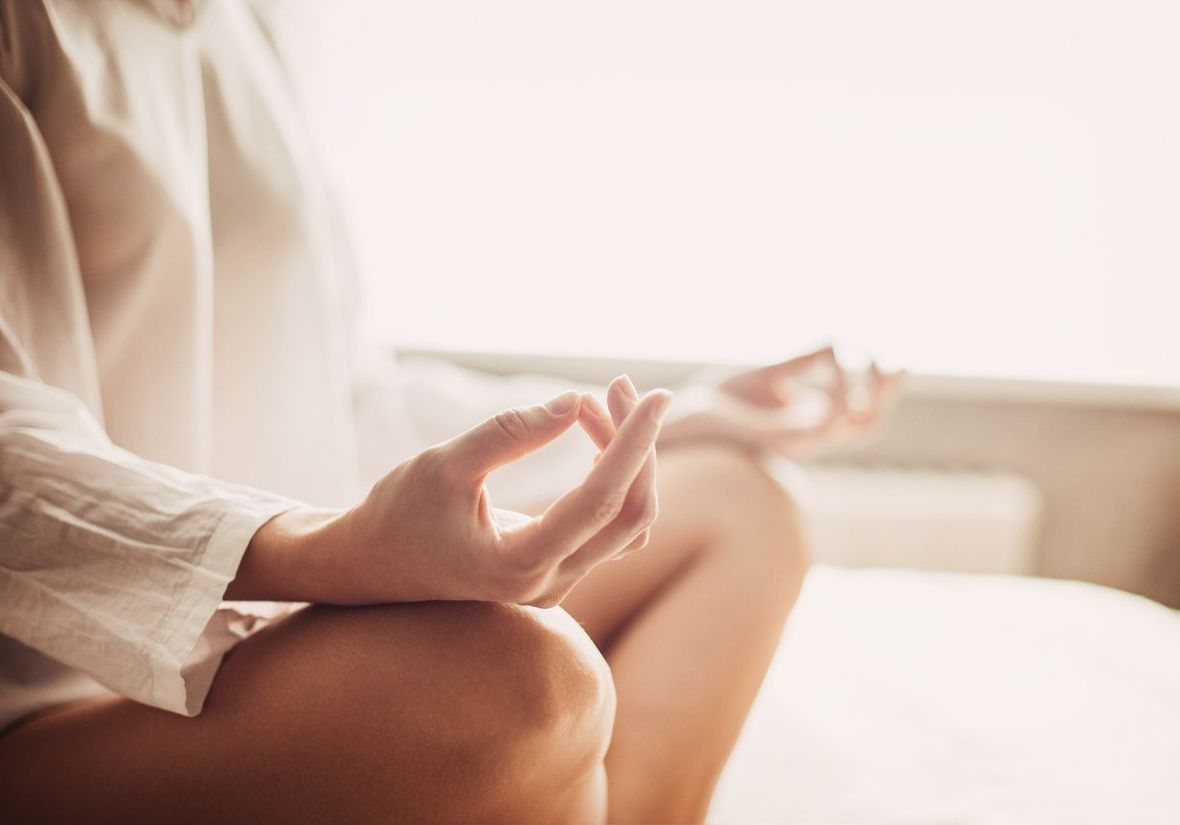## Private Sessions at the Yoga Atelier

You are able to book Private Sessions at the Yoga Atelier.

You have the option for a Solo or even book a group up to 4 for a Quartet Session!

Choose from the times below! Your First Private is 150.- after that you can book Care Packages that completely fit your needs and wants!

Monday - Friday
06:00 - 8:30

Monday
11:00 - 12:00
15:00 - 18:00

Tuesday
06:00 - 21:00

Wednesday
12:00 - 16:00

Friday
15:00 - 18:00

Saturday
09:00 - 12:00

 table div table+table+table+table+table+table+table+table+table+table+table+table+table+table+table+table+table div table{width:100%;padding:0}table div table+table+table+table+table+table+table+table+table+table+table+table+table+table+table+table+table div table img{width:96.23%;padding:0;float:none}table div table+table+table+table+table+table+table+table+table+table+table+table+table+table+table+table+table div table td{width:100%;padding:0 1.88% 18px}/* styles */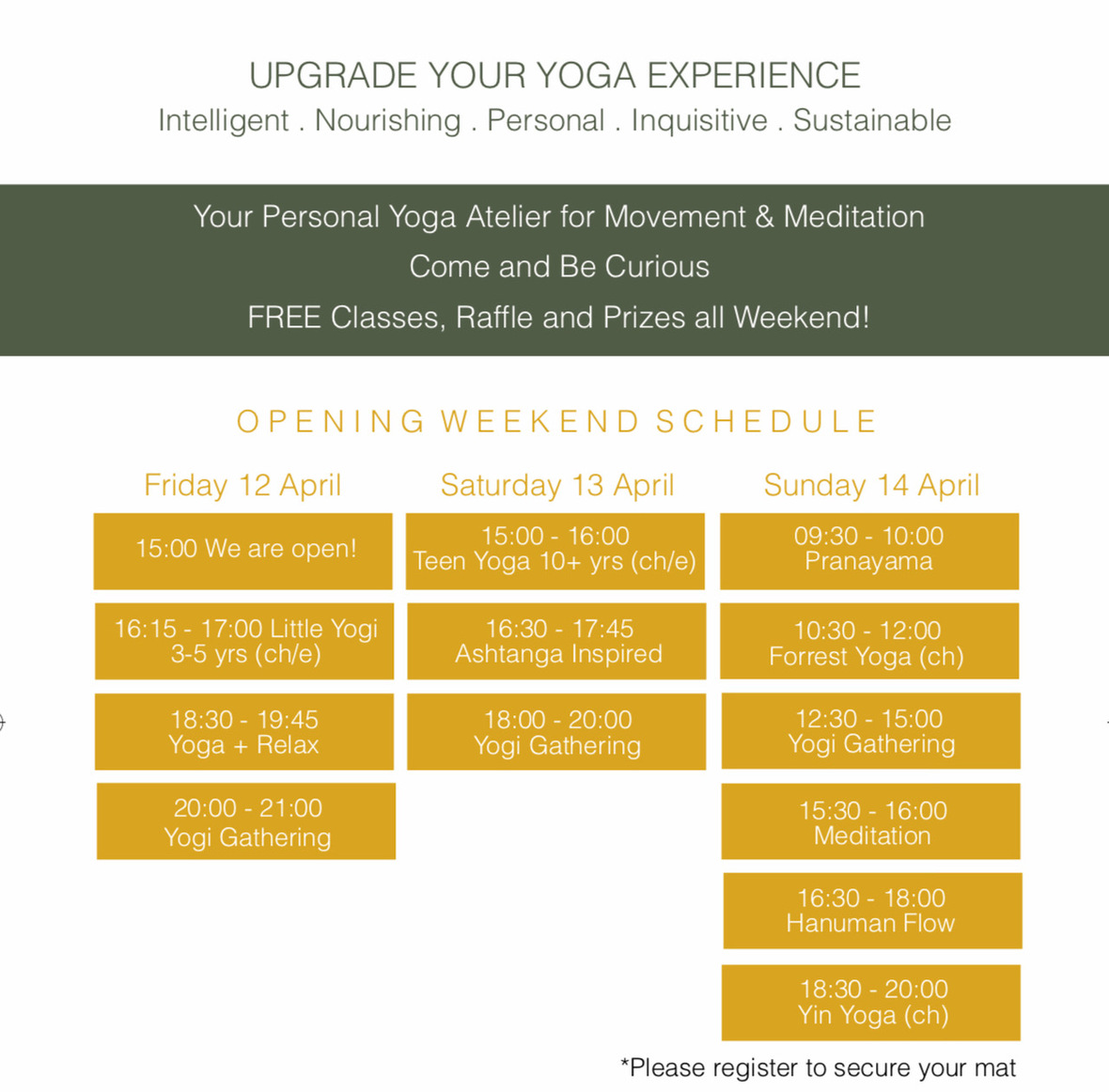## APRIL 12 - 14 | Celebration

Come and celebrate the Yoga Atelier's official opening with me!
l will teach free classes all weekend long! You will also get a chance to meet and take classes with Pascale! She will be a regular teacher at the Atelier! There is a suggested donation for each class that you can support Hirnliga or Krebsliga.

Check out the schedule on my website or on Facebook!

Make sure to register!
Register: yoga@chantalhauser.com
Location: Yoga Atelier, Florastrasse 13, 8800 Thalwil

 table div table+table+table+table+table+table+table+table+table+table+table+table+table+table+table+table+table+table+table+table div table{width:100%;padding:0}table div table+table+table+table+table+table+table+table+table+table+table+table+table+table+table+table+table+table+table+table div table img{width:96.23%;padding:0;float:none}table div table+table+table+table+table+table+table+table+table+table+table+table+table+table+table+table+table+table+table+table div table td{width:100%;padding:0 1.88% 18px}/* styles */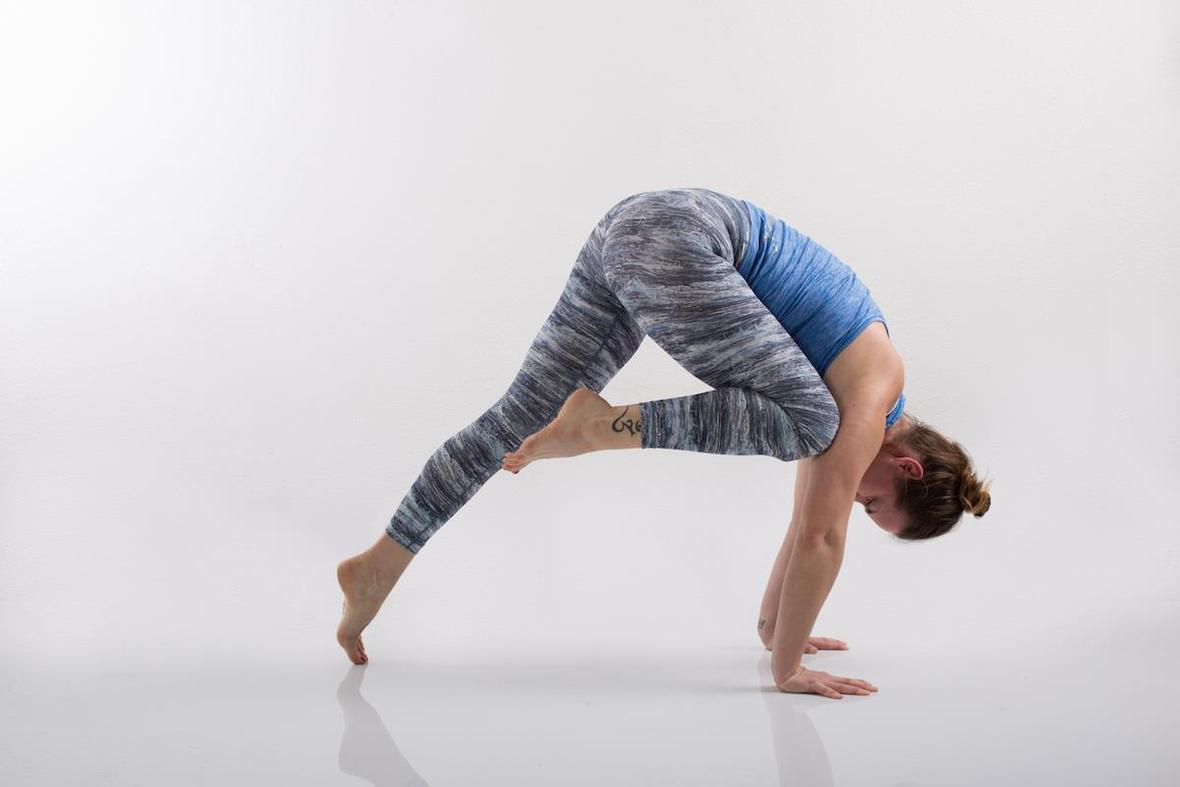## May 11 | Saturday Practice + Meditation

Practice with me on Saturday in Zurich! I am teaching Practice + Meditation and our focus will be on Inversions!

Time: 10:00 - 11:45
Location: The Space, Josefstrasse 130 in Zürich | K5
Invest: 45.- chf
Register: yoga@chantalhauser.com

 table div table+table+table+table+table+table+table+table+table+table+table+table+table+table+table+table+table+table+table+table+table+table+table div table{width:100%;padding:0}table div table+table+table+table+table+table+table+table+table+table+table+table+table+table+table+table+table+table+table+table+table+table+table div table img{width:96.23%;padding:0;float:none}table div table+table+table+table+table+table+table+table+table+table+table+table+table+table+table+table+table+table+table+table+table+table+table div table td{width:100%;padding:0 1.88% 18px}/* styles */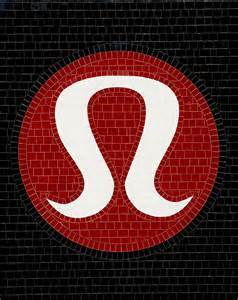## May 11 | Saturday lululemon Apero + Yogi Gathering

Join me after our Practice and Meditation session for a Yogi Gathering at lululemon in the city. Bring something to eat, I will provide snacks and coconut water too! Let's celebrate the opening of the Yoga Atelier and our yoga practice together!

Time: 13:00 - 14:30
Location: lululemon Zurich, Marktgasse 12 in Zürich | Niederdorf
Invest: Free
Register: yoga@chantalhauser.com

 table div table+table+table+table+table+table+table+table+table+table+table+table+table+table+table+table+table+table+table+table+table+table+table+table+table div table{width:100%;padding:0}table div table+table+table+table+table+table+table+table+table+table+table+table+table+table+table+table+table+table+table+table+table+table+table+table+table div table img{width:96.23%;padding:0;float:none}table div table+table+table+table+table+table+table+table+table+table+table+table+table+table+table+table+table+table+table+table+table+table+table+table+table div table td{width:100%;padding:0 1.88% 18px}/* styles */## More Happenings

May 12 | Sunday Mother's Day Events

14:00 - 15:00 Mom + Me Yoga
Kids 6+ come to Yoga with their Mom and get a take home snack and present

16:30 - 20:30 Mother's Day Yoga Apéro
A 90 minute class followed by food and a women's circle to celebrate motherhood

June 21 | Friday
Summer Solstice Outdoor Event in Thalwil

Early Summer | Forrest Yoga Weekend
at Davos Yoga in the Swiss Moutains

July 6 + August 10 | Saturday
Yoga Event at Yoga Scheune in Meilen

August 30 | Friday
End of Summer Fire Ceremony at Yoga Scheune in Meilen

 table div table+table+table+table+table+table+table+table+table+table+table+table+table+table+table+table+table+table+table+table+table+table+table+table+table+table+table div table{width:100%;padding:0}table div table+table+table+table+table+table+table+table+table+table+table+table+table+table+table+table+table+table+table+table+table+table+table+table+table+table+table div table img{width:96.23%;padding:0;float:none}table div table+table+table+table+table+table+table+table+table+table+table+table+table+table+table+table+table+table+table+table+table+table+table+table+table+table+table div table td{width:100%;padding:0 1.88% 18px}/* styles */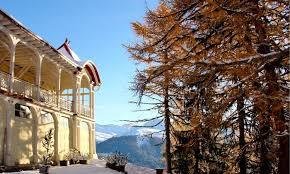## A Weekend Away in Davos

October 17 - 20
Nourish Your Soul - Yoga Weekend (E)

It is never too early to make October plans!
Join me on the Magic Mountain in Davos in the fall for a rejuvenating weekend away! You have the option to bring your partner and your babies too!

at Hotel Schatzalp in Davos | Magic Mountain | Facebook Event
Register with me: chantalhauseryoga@gmail.com

 table div table+table+table+table+table+table+table+table+table+table+table+table+table+table+table+table+table+table+table+table+table+table+table+table+table+table+table+table+table+table div table{width:100%;padding:0}table div table+table+table+table+table+table+table+table+table+table+table+table+table+table+table+table+table+table+table+table+table+table+table+table+table+table+table+table+table+table div table img{width:96.23%;padding:0;float:none}table div table+table+table+table+table+table+table+table+table+table+table+table+table+table+table+table+table+table+table+table+table+table+table+table+table+table+table+table+table+table div table td{width:100%;padding:0 1.88% 18px}/* styles */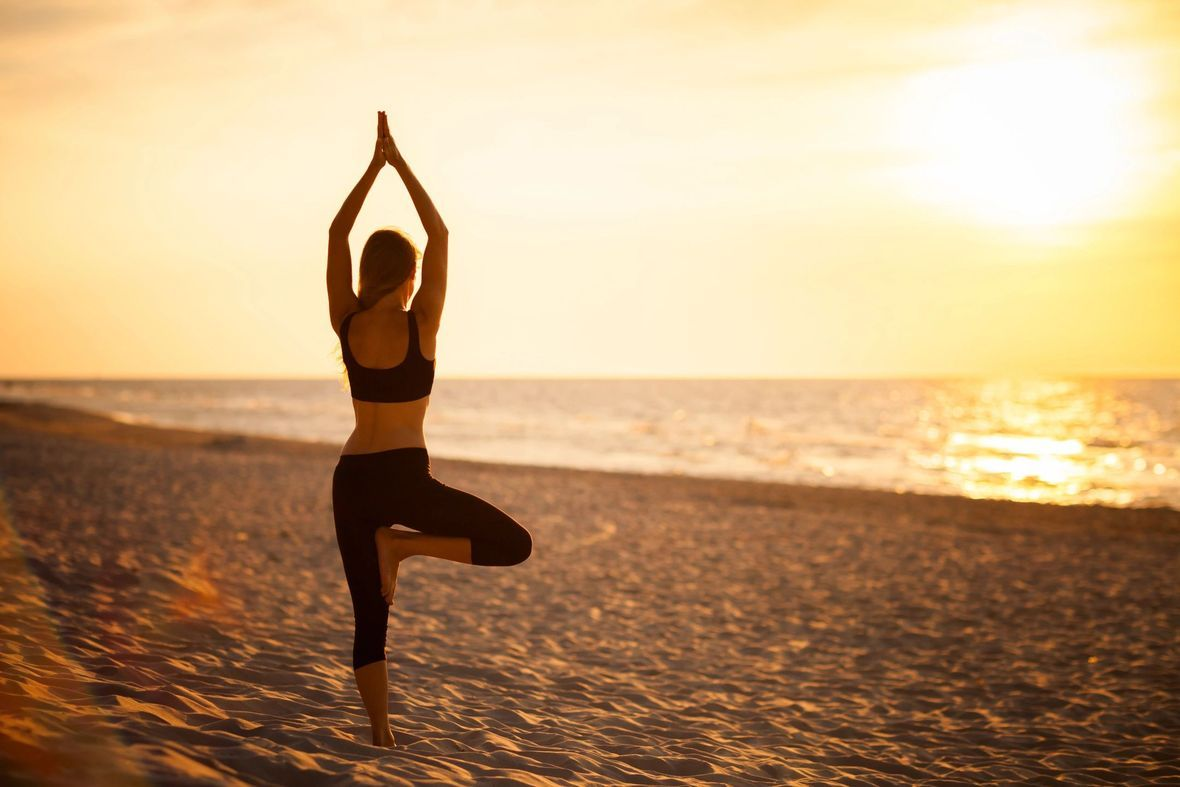What will Spring grow for me this year?

## Intention for March

Going with the Flow

Spring is usually a detoxifying and cleansing time. Spring Cleaning is in the air! It is time to charge your batteries for the Fire Season ahead. It is a great time to start your warm lemon water ritual in the morning and get some writing going before you do anything else. Even if it is only for 5 minutes! Write down whatever is in your head. Watch, you'll be amazed what you can put onto paper in the early mornings! (that is before even thinking about looking at your phone!) Go with the flow and see what happens!

Personally, I have been practicing a more flowing state of mind especially with how it relates to stress. It is definitely possible to move from a state of overwhelm to a state of movement. And isn't that the definition of yoga?
Patanjali wrote: Yogas Chitta Vritti Nirodha | We practice yoga to quiet the fluctuations of the mind.

 table div table+table+table+table+table+table+table+table+table+table+table+table+table+table+table+table+table+table+table+table+table+table+table+table+table+table+table+table+table+table+table+table+table div table{width:100%;padding:0}table div table+table+table+table+table+table+table+table+table+table+table+table+table+table+table+table+table+table+table+table+table+table+table+table+table+table+table+table+table+table+table+table+table div table img{width:96.23%;padding:0;float:none}table div table+table+table+table+table+table+table+table+table+table+table+table+table+table+table+table+table+table+table+table+table+table+table+table+table+table+table+table+table+table+table+table+table div table td{width:100%;padding:0 1.88% 18px}/* styles */table div table+table+table+table+table+table+table+table+table+table+table+table+table+table+table+table+table+table+table+table+table+table+table+table+table+table+table+table+table+table+table+table+table+table div table{width:100%;padding:0}table div table+table+table+table+table+table+table+table+table+table+table+table+table+table+table+table+table+table+table+table+table+table+table+table+table+table+table+table+table+table+table+table+table+table div table img{width:96.23%;padding:0;float:none}table div table+table+table+table+table+table+table+table+table+table+table+table+table+table+table+table+table+table+table+table+table+table+table+table+table+table+table+table+table+table+table+table+table+table div table td{width:100%;padding:0 1.88% 18px}/* styles */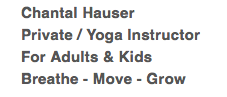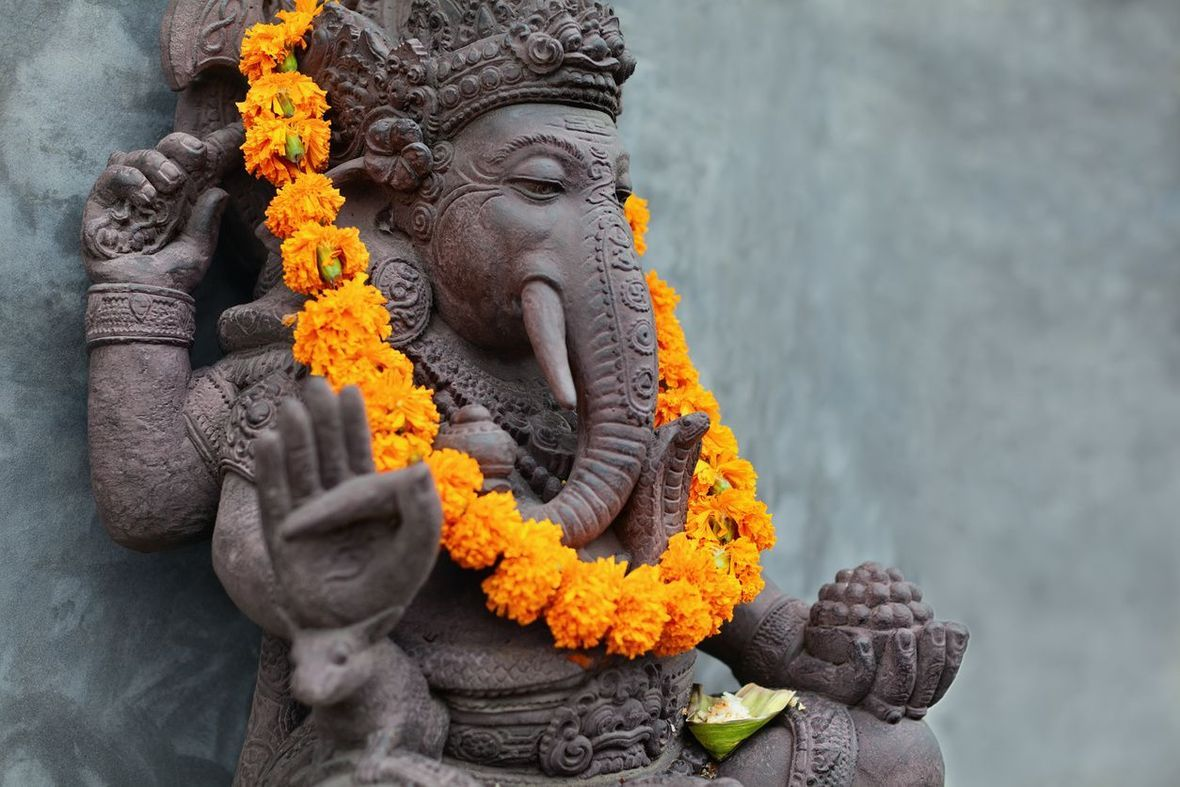table div table+table+table+table+table+table+table+table+table+table+table+table+table+table+table+table+table+table+table+table+table+table+table+table+table+table+table+table+table+table+table+table+table+table+table+table+table+table div table{width:100%;padding:0}table div table+table+table+table+table+table+table+table+table+table+table+table+table+table+table+table+table+table+table+table+table+table+table+table+table+table+table+table+table+table+table+table+table+table+table+table+table+table div table img{width:96.23%;padding:0;float:none}table div table+table+table+table+table+table+table+table+table+table+table+table+table+table+table+table+table+table+table+table+table+table+table+table+table+table+table+table+table+table+table+table+table+table+table+table+table+table div table td{width:100%;padding:0 1.88% 18px}/* styles */